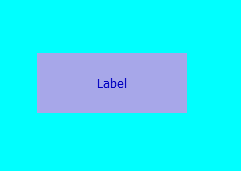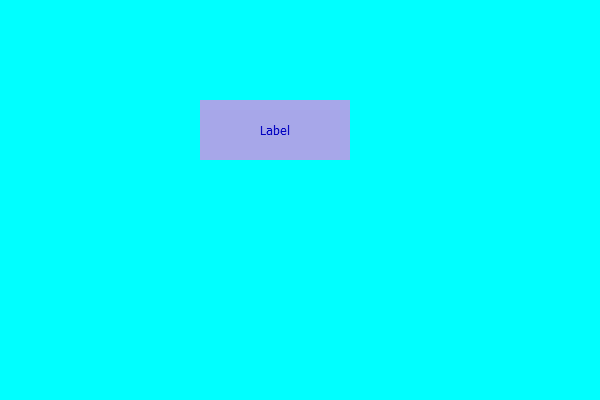# PyQt5 Label – Adding Color effect

• Last Updated : 10 May, 2020

In this article we will see how we can add color effect to the label by default there is no color effect to the label although we can create color effect for the label. Color effect is not like background color it is more like of colored filters we use on pictures, below is the representation of how label with color effect looks likeIn order to do this we have to do the following –

1. Create a label
2. Set geometry to the label
3. Create a QGraphicsColorizeEffect object
4. Add this object to the label with the help of setGraphicsEffect method

Syntax :

```# creating a color effect
color_effect = QGraphicsColorizeEffect()

# adding color effect to the label
label.setGraphicsEffect(color_effect)
```

Below is the implementation

 `# importing libraries``from` `PyQt5.QtWidgets ``import` `*` `from` `PyQt5 ``import` `QtCore, QtGui``from` `PyQt5.QtGui ``import` `*` `from` `PyQt5.QtCore ``import` `*` `import` `sys`` ` ` ` `class` `Window(QMainWindow):`` ` `    ``def` `__init__(``self``):``        ``super``().__init__()`` ` `        ``# maing background color cyan``        ``self``.setStyleSheet(``"background : cyan;"``)`` ` `        ``# setting title``        ``self``.setWindowTitle(``"Python "``)`` ` `        ``# setting geometry``        ``self``.setGeometry(``100``, ``100``, ``600``, ``400``)`` ` `        ``# calling method``        ``self``.UiComponents()`` ` `        ``# showing all the widgets``        ``self``.show()`` ` `    ``# method for widgets``    ``def` `UiComponents(``self``):`` ` `        ``# creating label``        ``label ``=` `QLabel(``"Label"``, ``self``)`` ` `        ``# setting geometry to the label``        ``label.setGeometry(``200``, ``100``, ``150``, ``60``)`` ` `        ``# setting alignment to the label``        ``label.setAlignment(Qt.AlignCenter)`` ` `        ``# creating a color effect``        ``color_effect ``=` `QGraphicsColorizeEffect()`` ` `        ``# adding color effect to the label``        ``label.setGraphicsEffect(color_effect)`` ` ` ` `# create pyqt5 app``App ``=` `QApplication(sys.argv)`` ` `# create the instance of our Window``window ``=` `Window()`` ` `# start the app``sys.exit(App.``exec``())`

Output :My Personal Notes arrow_drop_up